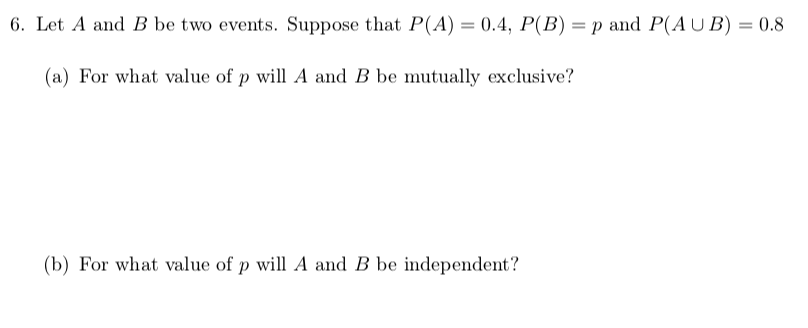# 6. Let A and B be two events. Suppose that P(A) = 0.4, P(B) = p and P(AUB) = 0.8(a) For what value of p will A and B be mutually exclusive?(b) For what value of p will A and B be independent?

Question
22 views

Question is attachedhelp_outlineImage Transcriptionclose6. Let A and B be two events. Suppose that P(A) = 0.4, P(B) = p and P(AUB) = 0.8 (a) For what value of p will A and B be mutually exclusive? (b) For what value of p will A and B be independent? fullscreen
check_circle

star
star
star
star
star
1 Rating
Step 1

(a)

Find the value of p for which A and B are mutually exclusive is obtained b...

### Want to see the full answer?

See Solution

#### Want to see this answer and more?

Solutions are written by subject experts who are available 24/7. Questions are typically answered within 1 hour.*

See Solution
*Response times may vary by subject and question.
Tagged in
MathProbability

### Basic Probability